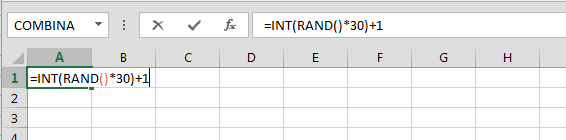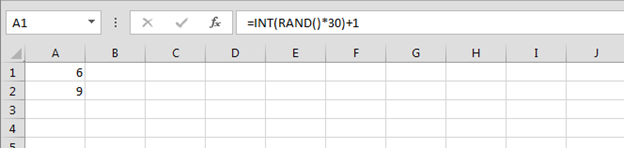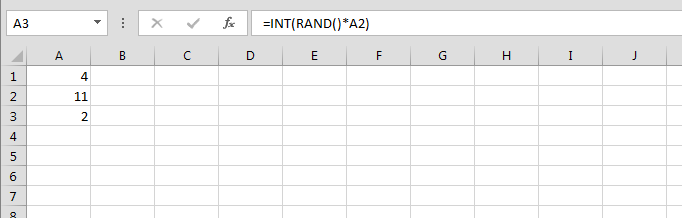Adjusting Values Returned by the RAND Function

In this chapter, you’ll learn about how to adjust values returned by RAND function. RAND function provide us the random numbers and it gets changed every time by doing any activity in the worksheet.

Let’s take an example and understand:

We want to retrieve two random numbers between 0 and 30, ensuring that the first number is always greater than the second one.

To solve this problem we will use INT function along with RAND function. You’ll learn two ways to use the combination of these formulas.

Follow below given steps and formulas:-

• Enter the formula in cell A1
• =INT(RAND()*30)+1• Copy the same formula in cell A2Let’s try another formula:-

• Enter the formula in cell A3
• =INT(RAND()*A2)By using functions this way you can get desired result in the cell. And, you can save lots of time.